# Ticks, tick labels, and grid lines¶

For the example in the following page we start from the example introduced in Initializing axes with world coordinates.

## Coordinate objects¶

While for many images, the coordinate axes are aligned with the pixel axes, this is not always the case, especially if there is any rotation in the world coordinate system, or in coordinate systems with high curvature, where the coupling between x- and y-axis to actual coordinates become less well-defined.

Therefore rather than referring to x and y ticks as Matplotlib does, we use specialized objects to access the coordinates. The coordinates used in the plot can be accessed using the coords attribute of the axes. As a reminder, if you use the pyplot interface, you can grab a reference to the axes when creating a subplot:

ax = plt.subplot()


or you can call plt.gca() at any time to get the current active axes:

ax = plt.gca()


If you use the object-oriented interface to Matplotlib, you should already have a reference to the axes.

Once you have an axes object, the coordinates can either be accessed by index:

lon = ax.coords
lat = ax.coords


or, in the case of common coordinate systems, by their name:

lon = ax.coords['glon']
lat = ax.coords['glat']


In this example, the image is in Galactic coordinates, so the coordinates are called glon and glat. For an image in equatorial coordinates, you would use ra and dec. The names are only available for specific celestial coordinate systems - for all other systems, you should use the index of the coordinate (0 or 1).

Each coordinate is an instance of the CoordinateHelper class, which can be used to control the appearance of the ticks, tick labels, grid lines, and axis labels associated with that coordinate.

## Axis labels¶

Axis labels can be added using the set_axislabel() method:

lon.set_axislabel('Galactic Longitude')
lat.set_axislabel('Galactic Latitude')


(png, svg, pdf)The padding of the axis label with respect to the axes can also be adjusted by using the minpad option. The default value for minpad is 1 and is in terms of the font size of the axis label text. Negative values are also allowed.

lon.set_axislabel('Galactic Longitude', minpad=0.3)


(png, svg, pdf)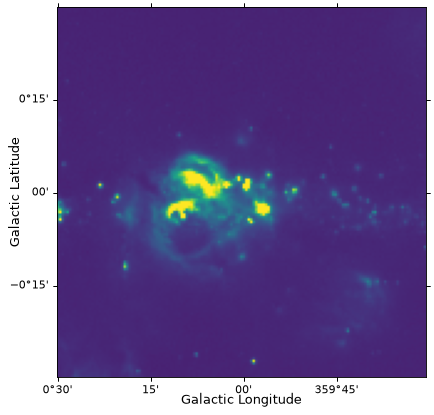Note

Note that, as shown in Getting started, it is also possible to use the normal plt.xlabel or ax.set_xlabel notation to set the axis labels in the case where they do appear on the x and y axis.

## Tick label format¶

The format of the tick labels can be specified with a string describing the format:

lon.set_major_formatter('dd:mm:ss.s')
lat.set_major_formatter('dd:mm')


(png, svg, pdf)The syntax for the format string is the following:

format

result

'dd'

'15d'

'dd:mm'

'15d24m'

'dd:mm:ss'

'15d23m32s'

'dd:mm:ss.s'

'15d23m32.0s'

'dd:mm:ss.ssss'

'15d23m32.0316s'

'hh'

'1h'

'hh:mm'

'1h02m'

'hh:mm:ss'

'1h01m34s'

'hh:mm:ss.s'

'1h01m34.1s'

'hh:mm:ss.ssss'

'1h01m34.1354s'

'd'

'15'

'd.d'

'15.4'

'd.dd'

'15.39'

'd.ddd'

'15.392'

'm'

'924'

'm.m'

'923.5'

'm.mm'

'923.53'

's'

'55412'

's.s'

'55412.0'

's.ss'

'55412.03'

'x.xxxx'

'15.3922'

'%.2f'

'15.39'

'%.3f'

'15.392'

'%d'

'15'

All the h..., d..., m..., and s... formats can be used for angular coordinate axes, while the x... format or valid Python formats (see String Formatting Operations) should be used for non-angular coordinate axes.

The separators for angular coordinate tick labels can also be set by specifying a string or a tuple.

lon.set_separator(('d', "'", '"'))
lat.set_separator(':-s')


(png, svg, pdf)## Tick/label spacing and properties¶

The spacing of ticks/tick labels should have a sensible default, but you may want to be able to manually specify the spacing. This can be done using the set_ticks() method. There are different options that can be used:

• Set the tick positions manually as an Astropy Quantity:

from astropy import units as u
lon.set_ticks([242.2, 242.3, 242.4] * u.degree)

• Set the spacing between ticks also as an Astropy Quantity:

lon.set_ticks(spacing=5. * u.arcmin)

• Set the approximate number of ticks:

lon.set_ticks(number=4)


In the case of angular axes, specifying the spacing as an Astropy Quantity avoids roundoff errors. The set_ticks() method can also be used to set the appearance (color and size) of the ticks, using the color= and size= options.

The set_ticklabel() method can be used to change settings for the tick labels, such as color, font, size, and so on:

lon.set_ticklabel(color='red', size=12)


In addition, this method has an option exclude_overlapping=True to prevent overlapping tick labels from being displayed.

We can apply this to the previous example:

from astropy import units as u
lon.set_ticks(spacing=10 * u.arcmin, color='white')
lat.set_ticks(spacing=10 * u.arcmin, color='white')
lon.set_ticklabel(exclude_overlapping=True)
lat.set_ticklabel(exclude_overlapping=True)


(png, svg, pdf)## Minor ticks¶

WCSAxes does not display minor ticks by default but these can be shown by using the display_minor_ticks() method. The default frequency of minor ticks is 5 but this can also be specified.

lon.display_minor_ticks(True)
lat.display_minor_ticks(True)
lat.set_minor_frequency(10)


(png, svg, pdf)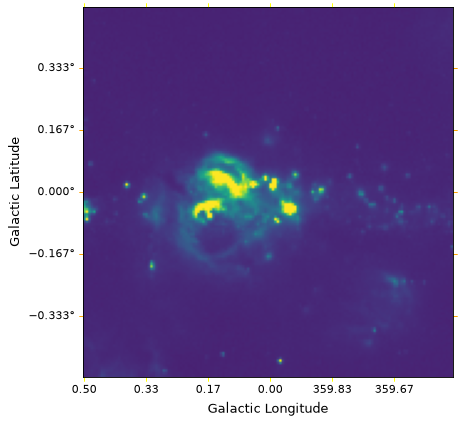## Tick, tick label, and axis label position¶

By default, the tick and axis labels for the first coordinate are shown on the x-axis, and the tick and axis labels for the second coordinate are shown on the y-axis. In addition, the ticks for both coordinates are shown on all axes. This can be customized using the set_ticks_position() and set_ticklabel_position() methods, which each take a string that can contain any or several of l, b, r, or t (indicating the ticks or tick labels should be shown on the left, bottom, right, or top axes respectively):

lon.set_ticks_position('bt')
lon.set_ticklabel_position('bt')
lon.set_axislabel_position('bt')
lat.set_ticks_position('lr')
lat.set_ticklabel_position('lr')
lat.set_axislabel_position('lr')


(png, svg, pdf)We can set the defaults back using:

lon.set_ticks_position('all')
lon.set_ticklabel_position('b')
lon.set_axislabel_position('b')
lat.set_ticks_position('all')
lat.set_ticklabel_position('l')
lat.set_axislabel_position('l')


(png, svg, pdf)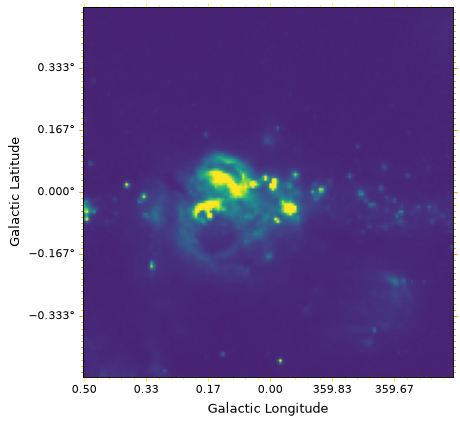On plots with elliptical frames, three alternate tick positions are supported: c for the outer circular or elliptical border, h for the horizontal axis (which is usually the major axis of the ellipse), and v for the vertical axis (which is usually the minor axis of the ellipse).

## Hiding ticks and tick labels¶

Sometimes it’s desirable to hide ticks and tick labels. A common scenario is where WCSAxes is being used in a grid of subplots and the tick labels are redundant across rows or columns. Tick labels and ticks can be hidden with the set_ticklabel_visible() and set_ticks_visible() methods, respectively:

lon.set_ticks_visible(False)
lon.set_ticklabel_visible(False)
lat.set_ticks_visible(False)
lat.set_ticklabel_visible(False)
lon.set_axislabel('')
lat.set_axislabel('')


(png, svg, pdf)And we can restore the ticks and tick labels again using:

lon.set_ticks_visible(True)
lon.set_ticklabel_visible(True)
lat.set_ticks_visible(True)
lat.set_ticklabel_visible(True)
lon.set_axislabel('Galactic Longitude')
lat.set_axislabel('Galactic Latitude')


(png, svg, pdf)## Coordinate grid¶

Since the properties of a coordinate grid are linked to the properties of the ticks and labels, grid lines ‘belong’ to the coordinate objects described above. For example, you can show a grid with yellow lines for RA and orange lines for declination with:

lon.grid(color='yellow', alpha=0.5, linestyle='solid')
lat.grid(color='orange', alpha=0.5, linestyle='solid')


(png, svg, pdf)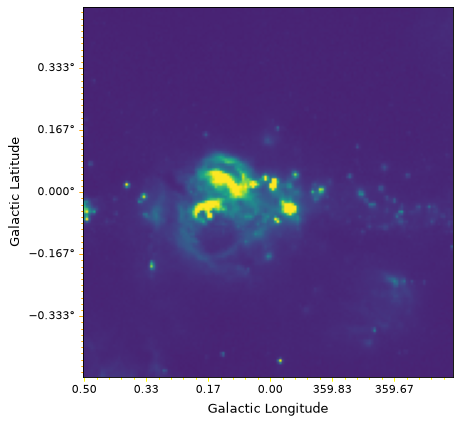For convenience, you can also simply draw a grid for all the coordinates in one command:

ax.coords.grid(color='white', alpha=0.5, linestyle='solid')


(png, svg, pdf)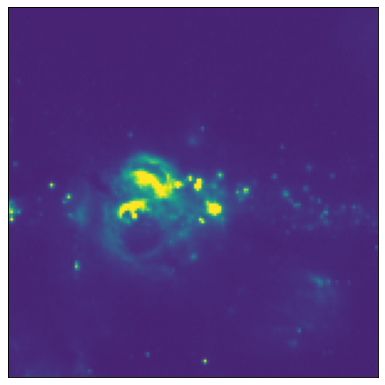Note

If you use the pyplot interface, you can also plot the grid using plt.grid().